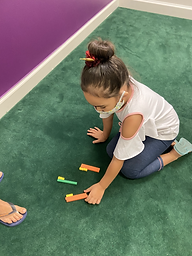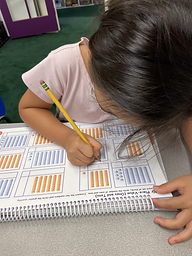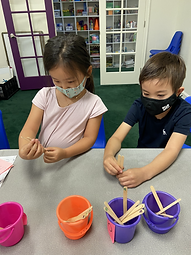## Ms. Joan

### Target 1​

###### Lesson Type:

Review

Number Operation

:

Number Reltionships

Compare numbers to determine which is more, less, or equal up to 50.

###### 1:

Compare quantities that can be counting to determine more, less, or equal.

###### 2:

Compare numerals to determine more, less, or equal.

###### 3:

Use manipulatives to determine more, less, or equal by equating quantity to length (i.e. Which is bigger 20 or 32? Line up 20 blocks end-to-end and 32 blocks end-to-end. Which is longer?).

Kindergarden

###### Vocabulary:

More, Less, Equal, Compare

Activities:

• Using base ten blocks, students were asked to compare two groups of blocks and identify if more, less or equal.
• Students were asked to put together 2 groups of blocks for peers and teacher to determine more, less or equal.### Home Exploration

###### Guiding Questions:## Absent Students:

### Target 2

:

###### 1:

Represent the composition or decomposition of two-digit numbers with an equation (13 = 10+3 or 1 ten and 3 ones).

Kindergarden

###### Vocabulary:

Tens place, Ones place

Activities:

• Using the tens base blocks, students were asked to identify which number is in tens and ones place.
• Students counted given groups of tens and ones to identify the given number. (eg. 5-tens 2-ones = 52)### Home Exploration

###### Guiding Questions:### Target 3

:

###### 1:

Determine whether to round up or down to get to the nearest ten.

###### 2:

Understand that the ones’ place is the key digit to evaluate to determine whether to round up or down.

###### 3:

Identify which digits in the ones’ place signal to round up to the nearest ten.

###### 4:

Identify which digits in the ones’ place signal to round down to the nearest ten.

Kindergarden

###### Vocabulary:

Round up, Round down

Activities:

• Students were asked to round up or down number from a popsicle stick (1-30) and numbered cups (0,10,20, 30).### Home Exploration